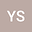Generating functions for series involving higher powers of inverse binomial coefficients and their applications
•## Abstract

The purpose of this paper is to construct generating functions in terms of hypergeometric function and logarithm function for finite and infinite sums involving higher powers of inverse binomial coefficients. These generating functions provide a novel way of examining higher powers of inverse binomial coefficients from the perspective of these sums, assessing how several of these sums and these coefficients related to each other. A unique relation between the Euler-Frobenius polynomial and B-spline associated with exponential Euler spline is reported. Moreover, with the aid of derivative operator and functional equations for generating functions, many new computational formulas involving the special finite sums of higher powers of (inverse) binomial coefficients, the Bernoulli polynomials and numbers, Euler polynomials and numbers, the Stirling numbers, the harmonic numbers, and finite sums are derived. Moreover, A few recurrence relations containing these particular finite sums are given. Using these recurence relations, we give a solution of the problem which was given by Charalambides7, Exercise 30, p. 273. We give calculations algorithms for these finite sums. Applying these algorithms and Wolfram Mathematica 12.0, we give some plots and many values of these polynomials and finite sums.
06 Feb 2023Submitted to Mathematical Methods in the Applied Sciences
06 Feb 2023Submission Checks Completed
06 Feb 2023Assigned to Editor
10 Feb 2023Review(s) Completed, Editorial Evaluation Pending
10 Feb 2023Reviewer(s) Assigned
20 Feb 2023Editorial Decision: Revise Minor